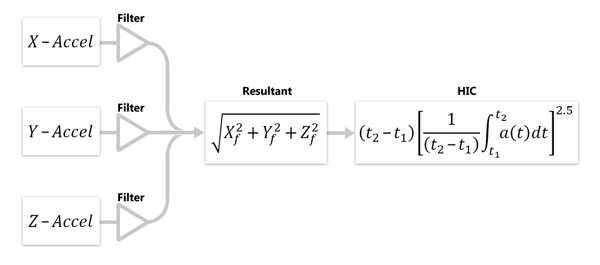# Tutorial 9: Page 5

Home » Knowledge Base » LS-Prepost » Tutorials » Extended Tutorials » Tutorial 9 » Tutorial 9: Page 5

• 5. Pre-filtering explained
• Figure 7 illustrates the concept of pre-filtering the acceleration signal before calculating HIC. The computation is performed as follows:

• 1. X, Y, and Z components of acceleration are filtered
• 2. Resultant acceleration is plotted
• 3. HIC is calculated

•• Figure 7